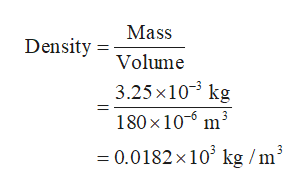# A solution is made by dissolving 3.25 grams of a substance in 180 mL of water. Calculate the density of the solution in kg/m3.

Question
2 views

A solution is made by dissolving 3.25 grams of a substance in 180 mL of water. Calculate the density of the solution in kg/m3.

check_circle

Step 1

The given mass is 3.25 g.

It is known that, 1 g = 10-3 kg.

Therefore, the value of mass in kg is 3.25×10-3.

The given volume is 180 mL.

It is known that 1 mL = 10-3 L

Therefore, volume in liter is 180×10-3.

The conversion of 1 L into m3 is as follows:

1 L = 10-3 m3

Therefore, the value of volume in m3 is 180×10-6.

Step 2

The formula to calculate density is as follows:

Step 3

Put all the values in the formu...help_outlineImage TranscriptioncloseMass Density Volume 3.25 x103 kg 180 x 10 m3 0.0182x 103 kg /m fullscreen

### Want to see the full answer?

See Solution

#### Want to see this answer and more?

Solutions are written by subject experts who are available 24/7. Questions are typically answered within 1 hour.*

See Solution
*Response times may vary by subject and question.
Tagged in

### Chemistry